Question

A mass MM uniform solid cylinder of radius RR and a mass MM thin uniform spherical shell of radius RR roll without slipping. If both objects have the same kinetic energy, what is the ratio of the speed of the cylinder to the speed of the spherical shell

1.hongcuc2

vcyl / vsph = 1.05

Explanation:

• The kinetic energy of a rolling object can be expressed as the sum of a translational kinetic energy plus a rotational kinetic energy.
• The traslational part can be written as follows: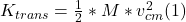• The rotational part can be expressed as follows: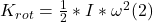• where I = moment of Inertia regarding the axis of rotation.
• ω = angular speed of the rotating object.
• If the object has a radius R, and it rolls without slipping, there is a fixed relationship between the linear and angular speed, as follows: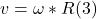• For a solid cylinder, I = M*R²/2 (4)
• Replacing (3) and (4)  in (2), we get: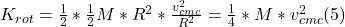• Adding (5) and (1), we get the total kinetic energy for the solid cylinder, as follows: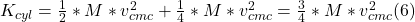• Repeating the same steps for the spherical shell: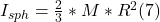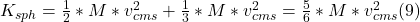• Since we know that both masses are equal each other, we can simplify (6) and (9), cancelling both masses out.
• And since we also know that both objects have the same kinetic energy, this means that (6) are (9) are equal each other.
• Rearranging, and taking square roots on both sides, we get: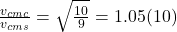• This means that the solid cylinder is 5% faster than the spherical shell, which is due to the larger moment of inertia for the shell.